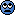# I Trying to obtain a 2nd order ODE

#### AAli

Hi I'm having a slight issue trying to obtain a 2nd order ODE with respect to x (so involves implicit differentiation in this case) from the equation below. I would greatly appreciate any help or tips to solve this problem.I've removed the coefficients to make things a litter easier. Thank you.

#### Attachments

• 2.3 KB Views: 538
Last edited by a moderator:
Related Differential Equations News on Phys.org

#### Mark44

Mentor
Hi I'm having a slight issue trying to obtain a 2nd ODE with respect to x (so involves implicit differentiation in this case) from the equation below. I would greatly appreciate any help or tips to solve this problem.

I've removed the coefficients to make things a litter easier. Thank you.
Your question isn't clear to me. Are you trying to obtain a 2nd order ODE? If so, I'm not sure that will lead to anything productive. A different approach would be to move all the terms involving dy/dx to one side, and then solve for dy/dx in terms of everything else. The resulting equation is separable, so it's possible a solution could be found without resorting to the implicit differentiation you're talking about.

#### AAli

Your question isn't clear to me. Are you trying to obtain a 2nd order ODE? If so, I'm not sure that will lead to anything productive. A different approach would be to move all the terms involving dy/dx to one side, and then solve for dy/dx in terms of everything else. The resulting equation is separable, so it's possible a solution could be found without resorting to the implicit differentiation you're talking about.
Hi, my goal was to eventually obtain a set of coupled first order differential equations from the 2nd order ODE, so I'm happy to try a quicker approach to solve this problem. Could you please elaborate on the highlighted bit. Thank you.

Edit: order

#### Mark44

Mentor
Hi, my goal was to eventually obtain a set of coupled first order differential equations from the 2nd ODE, so I'm happy to try a quicker approach to solve this problem. Could you please elaborate on the highlighted bit. Thank you.
"2nd ODE" does not mean "second order differential equation." Please try to be more clear in what you write. Edit: I see that you have edited your post to include the word "order."

The strategy you're describing won't work, as far as I can tell. It does no good to convert a 1st-order DE to 2nd order for the purpose obtaining two first-order DEs.
What I'm suggesting is to move all the terms in dy/dx to one side, and then solve algebraically for dy/dx.
I get $\frac {dy}{dx} = \frac{y^{1/2}(1 - y^{1/2} - y)}{y^{1/2}(y^{-1/2} + 1 - y)}$.
This is separable, and would be very simple to solve except for one sign in the fraction on the right.

•AAli

#### AAli

"2nd ODE" does not mean "second order differential equation." Please try to be more clear in what you write. Edit: I see that you have edited your post to include the word "order."

The strategy you're describing won't work, as far as I can tell. It does no good to convert a 1st-order DE to 2nd order for the purpose obtaining two first-order DEs.
What I'm suggesting is to move all the terms in dy/dx to one side, and then solve algebraically for dy/dx.
I get $\frac {dy}{dx} = \frac{y^{1/2}(1 - y^{1/2} - y)}{y^{1/2}(y^{-1/2} + 1 - y)}$.
This is separable, and would be very simple to solve except for one sign in the fraction on the right.
I did as you advised but I got something slightly different:

$\frac {dy}{dx} = \frac{y^{1/2}(1 - y^{1/2} - y)}{y^{1/2}(y^{-1/2} + 1 - y^{-3/2})}$.

#### Mark44

Mentor
I did as you advised but I got something slightly different:

$\frac {dy}{dx} = \frac{y^{1/2}(1 - y^{1/2} - y)}{y^{1/2}(y^{-1/2} + 1 - y^{-3/2})}$.
Yes, I made a mistake on the last term in the denominator.

You mentioned earlier that you removed the coefficients. You should put them back in, as both numerator and denominator might be further factorable.

#### epenguin

Homework Helper
Gold MemberIsn't it just dy/dx = - y. ?

### Want to reply to this thread?

"Trying to obtain a 2nd order ODE"

### Physics Forums Values

We Value Quality
• Topics based on mainstream science
• Proper English grammar and spelling
We Value Civility
• Positive and compassionate attitudes
• Patience while debating
We Value Productivity
• Disciplined to remain on-topic
• Recognition of own weaknesses
• Solo and co-op problem solving